# Dynamic Problem Maker

No two assignments should ever be the same.
App Type: Homework or Assessment Creator
Best For: Creating Word Problems
WHY
PART 1
PART 2
PART 3
PART 4
PART 5
EXAMPLE

WHY:
Most worksheets and even online website problems are static, or they're drawn from a question bank. What if we could create problems where the numbers are dynamically changing each time a student does the same problem?

The Problem: Achieving this requires a teacher to know how to code to create such problems.
The Solution: A programmer can create a program that will enable teachers to create those dynamically changing problems. In fact, the problem can even grade itself!

INSTANT FEEDBACK:
Students need instant feedback to do well. The traditional form of homework is to pass it out on Monday, collect it on Tuesday, and hand it back on Wednesday. A student will find out whether they understood Monday's lesson on Wednesday. Imagine a coach observing an athlete and telling that athlete, "I'll tell you in 2 days what you did wrong." The problems generated using the Dynamic Problem Maker is programmed to give students instant feedback, telling a student whether the answer is right or wrong, if it's missing units, and even provide a link to the lesson.

TEACHING EACH OTHER:
The days of students asking each other "What's your answer for number..." is over. Whether the student was checking an answer with a friend or simplying copying, they cannot simply just give answers. While the questions are the same, the numbers are different; therefore, students will have to show or teach each other how to solve each problem.

STUDY TOOL:
Studying the same problems that have been assigned for homework may work, but students often complain that they already did them or that they already know the answers to them. With dynamic problems, students can click RESET and a new problems with new numbers will be dynamically generated.

FOCUS ON DEEPER LEARNING CONCERNS:
Since students can't simply copy each other 5 minutes before class, students who are struggling to grasp concepts are easier to target. Students can't simply "get by" with turning in homework only for a teacher to find out after an assessment that the student didn't actually understand the concepts. Focusing energy on identifying students who are struggling is better than continuous grading.

What seperates privileged and under-priviledged students is access to resources like private tutoring. Private tutors instantly show a student how to do something when they're having difficulties. While not perfect, an alternative is linking resources right on the homework so that a student can instantly get help.

### PART I - Basic Information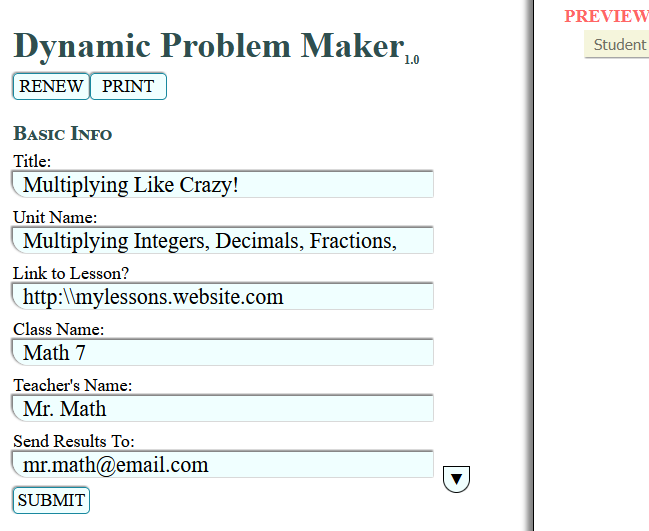TEACHER'S GUIDE:
Fill in the information you would normally put on any other assignments. Here, you can also type in a link to a lesson or video that will help students in case they need help with the work.

After clicking submit, you will see the information populated on the "Preview Page". You can always click EDIT to edit the information.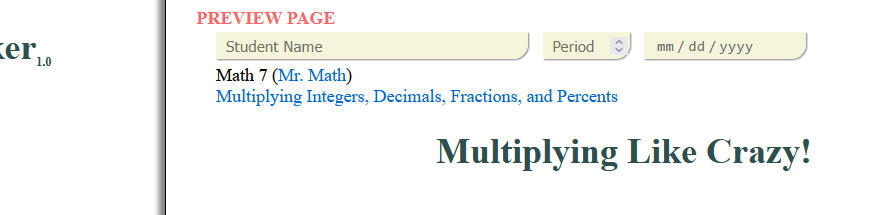### PART II - Create the Problem

TEACHER'S GUIDE: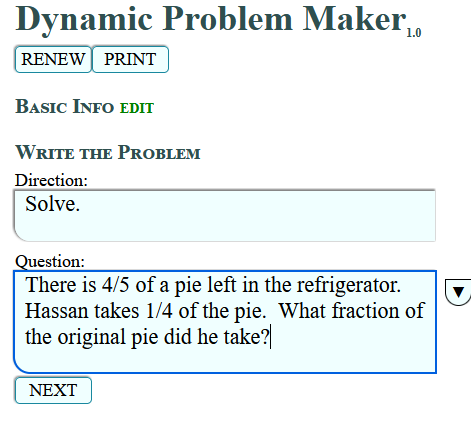Write the directions and an engaging word problem. The progam will identify all the numbers written in the problem and create variables out of them. A little knowledge of Algebra will go a long way! Use the pop up tool to add squares and cubes to units (i.e. - cm², m³), pi (π), and degreees (°).

**Note** The program does not recognize radicals (i.e. - square roots). When typing exponents (not on units), use the caret (^). Example 5^2 will be converted to $5^2$.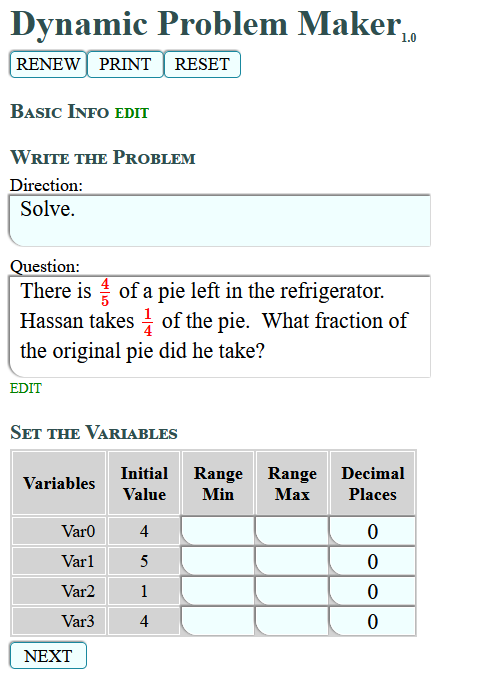### PART III - Set the Variables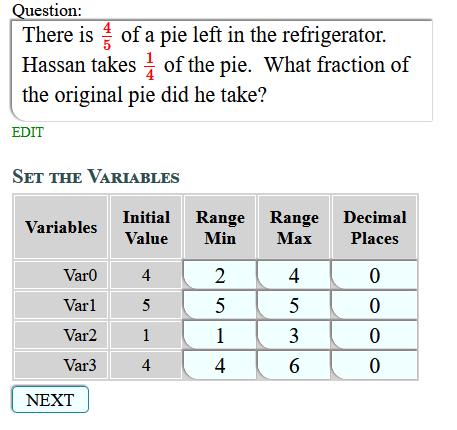TEACHER'S GUIDE:
To dynamically create random numbers in your problem, you'll have to give each number a realistic range. If you want certain numbers to be static use the same number for the "min" and "max". You can also designate how many decimal spaces you'd like each number to dynamically generated. The program will try to recognize this based on your typed problem from the previous step.

In this example, the numerator of the first fraction has a range of 2 to 4, while the denominator will remain static at 5. The second fraction will have a dynamic range for both the numerator and the denominator.

### PART IV - Formulate the Solution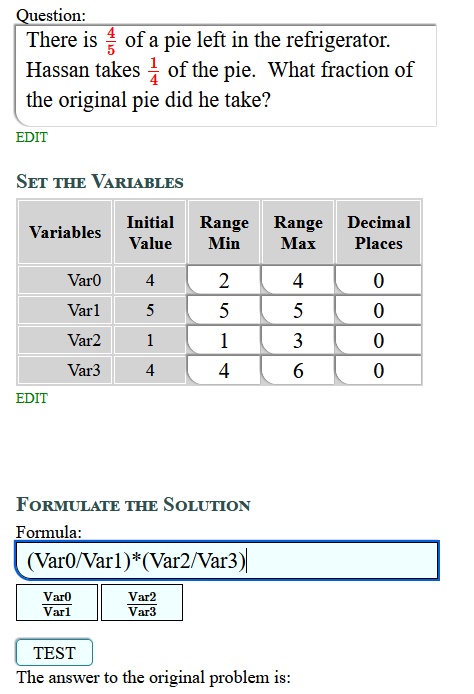TEACHER'S GUIDE:
You'll have to know some very basic Algebra here. Basically if the problem requires that the first number is to be multiplied with the second number, then your formula is "Var0*Var1". That then, will be how every problem will be solved regardless of the numbers that are generated. Variable buttons are created for you to make things easier, especially with fractions and exponents.

Test your formula and select whether you want your answer as a fraction or a decimal. You can designate improper or mixed fractions by clicking on the fraction button repeatedly. You can also designate the number of decimal spaces to round to for your answer. In addition, you can ask for all answers to always be rounded up (for problems like "How many pencils will Jerome need to buy?") or always round down (for problems like "How many can Katie afford to buy?").

In addition, if your problem has fractions, you may ask the program to generate only problems with whole number answers. While the problem will still generate dynamic fractions, all answers will be whole number answers.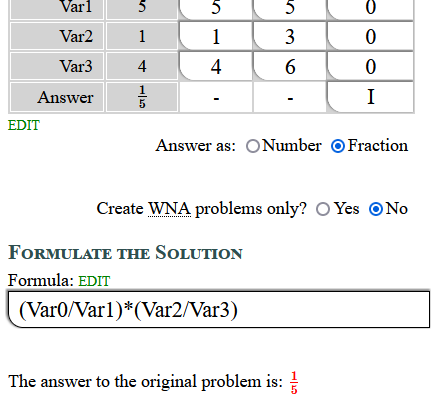Remember to always check to see if the answer to your question (in red) is correct. This means that you created the correct formula! If not, go back and edit the formula and test it again.

### PART V - Format the Answer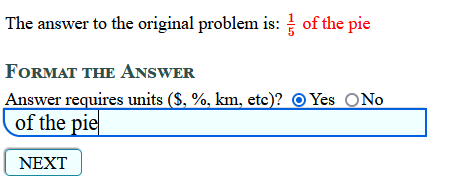TEACHER'S GUIDE:
Most word problems will require units. Students will be required to type in units with their answers in order to get a problem correct. However, the program will recognize that a problem may be correct numerically but simply missing units or if the student typed an incorrect unit.

After clicking next, and if all has been entered without error, you will see the green button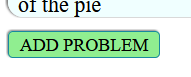.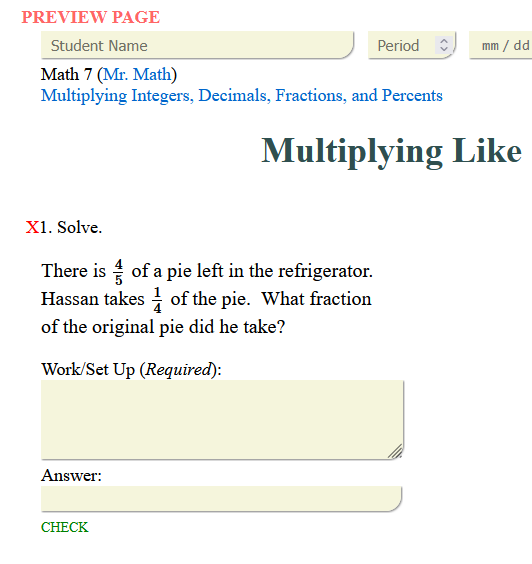Clicking this will add the problem onto the PREVIEW PAGE. The left hand side will reset for you to create your next dynamic problem. When you're all done, you can click on the PRINT button on top and then DOWNLOAD the file (as an html file).

This is an example of a completed dynamic problem worksheet. If you refresh the browser, you should see that the numbers in the problem dynamically change. Therefore, it is very unlikely that two students will have the same worksheet.